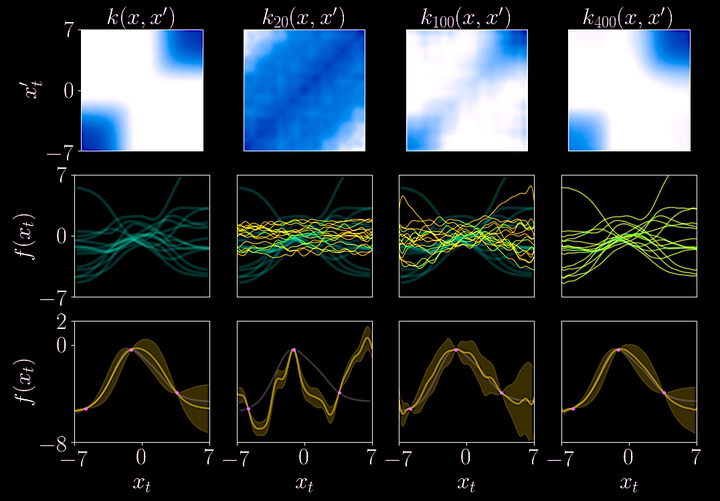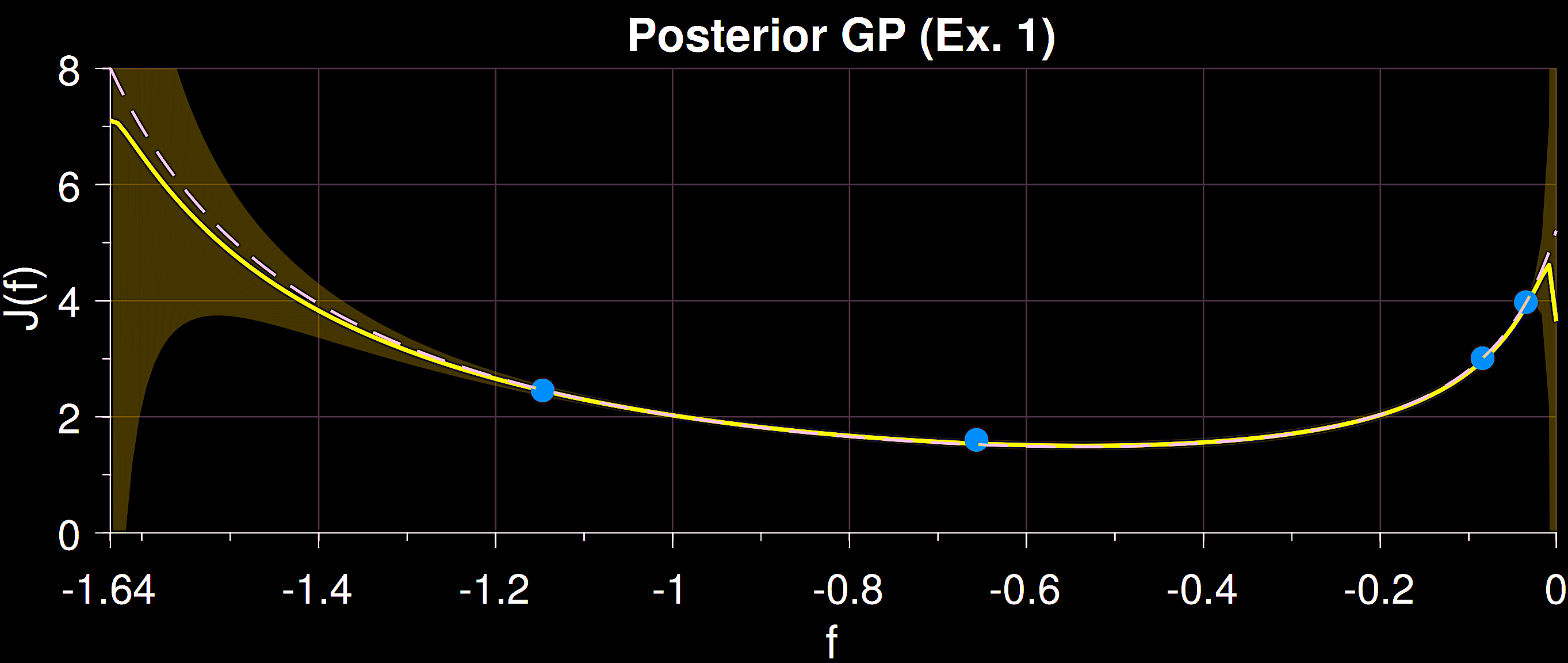# Increasing Data Efficiency in model-based RL via Informed Probabilistic PriorsFigure 1
Controlling highly complex dynamical systems, such as walking quadrupeds, is particularly challenging in the absence of an accurate representation of the dynamics. Herein, we represent the dynamics using probabilistic models, able to capture such epistemic uncertainty in form of a posterior distribution. In particular, we focus on Bayesian neural networks (BNN) and Gaussian processes (GPs) to model state-space trajectories over a certain time horizon.

Even though GPs and BNN are universal function approximators, they are hindered by low learning rates when the hypothesis space of the prior is too wide. This can be mitigated by injecting structure or information into the prior, hence shrinking the hypothesis space and increasing such learning rates. For certain dynamical systems, like walking robots, we usually have access to some form of information about the rigid body dynamics, e.g., first principles dynamical equations or high fidelity simulators. In the following, I describe two projects where I have explored these ideas.

## LQR kernel design for automatic controller tuningFigure 2. A Gaussian process (yellow) with an informed LQR kernel models accurately the true cost function (dashed line) from only 4 observations.In , we proposed an entry-level attack to this problem. Therein, the goal is to automatically learn the parameters of a linear quadratic regulator (LQR) by iteratively minimizing the cost of the real system tracking an equilibrium point. The learning algorithm is realized with Bayesian optimization, which iteratively selects the most informative controller parameters at each iteration. The uncertainty about the dynamical system propagates to the performance metric, which is modeled as a Gaussian process. In order to speed up the learning process, we construct the kernel by using a closed loop model of the true system with uncertain parameters. This kernel aids the GP to extrapolate more accurately in regions where we have no data (see Fig. 1) Our results show that the proposed LQR kernel outperforms standard kernels that are not informed with the system dynamics.

## Designing informed kernels for learning dynamical systems

In , the goal is to represent a nonlinear dynamical system using multi-output Gaussian process with an informed kernel. Such kernel is constructed using simulated state trajectories by means of the Fourier transform of the dynamics. The resulting model captures the dynamics of the simulator, while also providing an explicit representation of the epistemic uncertainty about the true dynamics. Our experiments show that this kernel helps to mitigate the “sim2real” gap, while outperforming standard kernels that are not informed with the dynamics.

The kernel is constructed using a set of features, which are nonlinear mappings from Fourier domain to the state space domain. We need an increasing number of features to meaningfully represent the sought kernel. We illustrate this in Fig. 1 (top row) where the a groundtruth kernel of a scalar system is reconstructed from simulated system draws. As the number of features increases, the sampled functions are better captured (middle row). When such kernel is used for GP regression, the larger the number of features, the better is the GP able to capture the true function (bottom row).

 On the Design of LQR Kernels for Efficient Controller Learning
A. Marco, P. Hennig, S. Schaal, and S. Trimpe
Proceedings of the 56th IEEE Annual Conference on Decision and Control (CDC), Dec. 2017, pp. 5193–5200
[paper] [presentation]

 Out of Distribution Detection via Domain-Informed Gaussian Process State Space Models
A. Marco, Elias Morley, Claire J. Tomlin Proceedings of the 56th IEEE Annual Conference on Decision and Control (CDC), Dec. 2023 (under review)